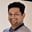Related Tags

sqrt
java
binary search
square root
communitycreator

# How to find the square root of an integer using binary searchTarun Telang

## Problem

Given a positive integer (N), find the square root of the number using binary search.

## Algorithm

Below are the steps to find the square root of an integer (N) using binary search.

Step 1: Let Left = 1, and Right = N.

Step 2: Loop until Left <= Right

Step 2.1: Middle = (Left + Right ) / 2, Square = Middle * Middle.

Step 2.2: If (Square == N), return Middle as the answer.

Step 2.3: If (Square < N), then Left = Middle + 1, go to Step 1.

Step 2.4: Else Right = Middle - 1 (as Square > N)

Step 2.5: Go to Step 1.

Step 3: Return NOT_A_PERFECT_SQUARE (which in this case would be a -1 because the function has to return an integer).

Take a look at the code below to understand this better.

## Code

In our code, we utilize BigInteger class to store large squared numbers that overflow if we use int data type.

import java.math.BigInteger;
class SquareRoot {
public static final BigInteger NOT_A_PERFECT_SQUARE = new BigInteger("-1");
public static BigInteger squareRoot(long n){
/*Base condition when n=0 because for this case, left would
be greater than the right and sqaure root wouldn't be computed
for 0 and -1 will b returned.*/
if (n==0)
{
return BigInteger.valueOf(0);
}
// For n > 0
BigInteger left = new BigInteger("1");
BigInteger right = BigInteger.valueOf(n);
BigInteger middle = new BigInteger("1");
while((left.compareTo(right)) == 0 || (left.compareTo(right)) == -1) {
BigInteger square = middle.multiply(middle);
int check1 = square.compareTo(BigInteger.valueOf(n));
if (check1==0) {
return middle;
}
if (check1 == -1) {
} else {
right = middle.subtract(BigInteger.valueOf(1));
}
}
return NOT_A_PERFECT_SQUARE;
}

public static void main( String args[] ) {
System.out.println( "Square root of 24 : " + squareRoot(24));
System.out.println( "Square root of 1: " + squareRoot(1));
System.out.println( "Square root of 4: " + squareRoot(4));
System.out.println( "Square root of 9: " + squareRoot(9));
System.out.println( "Square root of 16: " + squareRoot(16));
System.out.println( "Square root of 25: " + squareRoot(25));
System.out.println( "Square root of 36: " + squareRoot(36));
System.out.println( "Square root of 49: " + squareRoot(49));
System.out.println( "Square root of 64: " + squareRoot(64));
System.out.println( "Square root of 81: " + squareRoot(81));
System.out.println( "Square root of 100: " + squareRoot(100));
}
}

Square Root

## Conclusion

The time complexity of this algorithm is O(log(N)), logarithmic time. Here, N is the number for which the square root is to be computed. The space complexity of the solution is O(log(1), constant space. This is because we don’t need any additional storage other than local variables like left, right, middle, and square.

RELATED TAGS

sqrt
java
binary search
square root
communitycreator

CONTRIBUTORTarun Telang
RELATED COURSES

View all Courses

Keep Exploring

Learn in-demand tech skills in half the time